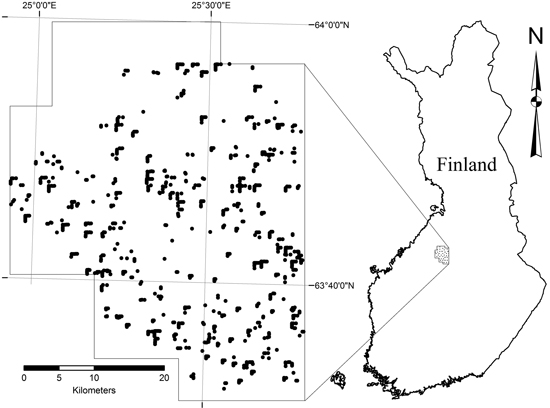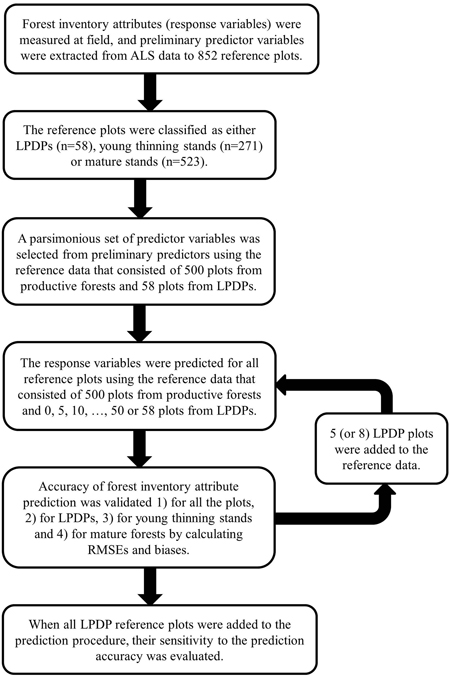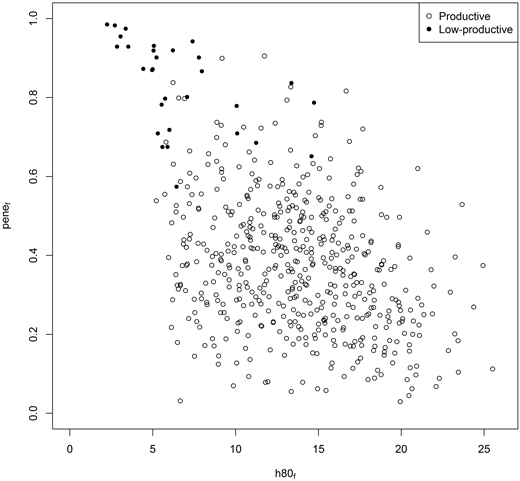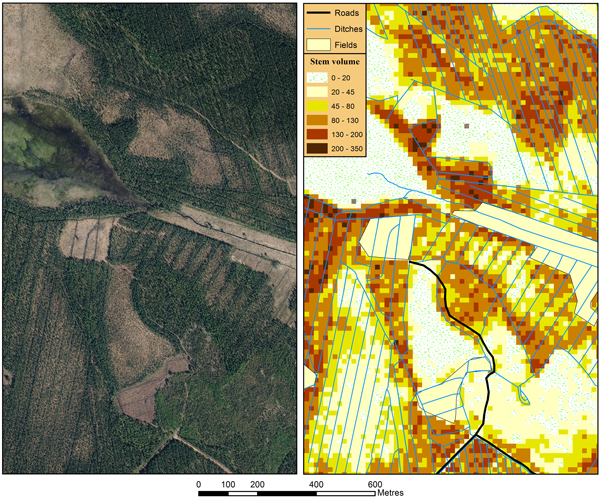Fig. 1. ALS data coverage and sample plot location.

 Table 1. Predictor candidates (n = 38) derived from ALS data. Variable name Variable description h10f – h100f Point height at the r percentile of the first-echo points’ height distribution (r = 10, 20... 100); ground points, i.e. points below the ground threshold (= 2 m), are excluded. h10l – h100l Point height at the r percentile of the last-echo points’ height distribution (r = 10, 20... 100); ground points are excluded. penef Penetration of first-echo points; calculated as the ratio of the number of ground points to the number of all points penel Penetration of last-echo points HVmean The mean of the first-echo high-vegetation points, i.e. points above the high vegetation threshold (= 5 m) HVsd Standard deviation of the Z coordinates for the high vegetation first-echo points pc1l – pc8l Ratio of the number of last-echo points with heights smaller or equal to hmax to the number of all last-echo points, where hmax = hclassstart + i * hclasssize (i = 1, 2… 8; hclassstart = 1.5 m; hclasssize = 2.0 m) p8530f Proportional canopy density at a height equalling 30% of the 85th percentile of first-echo points’ height distribution; calculated as the ratio of the number of first-echo points below that height to the number of all first-echo points p8580f Proportional canopy density at a height equalling 80% of the 85th percentile of first-echo points’ height distribution p9030f Proportional canopy density at a height equalling 30% of the 90th percentile of first-echo points’ height distribution p9080f Proportional canopy density at a height equalling 80% of the 90th percentile of first-echo points’ height distribution p9530f Proportional canopy density at a height equalling 30% of the 95th percentile of first-echo points’ height distribution p9580f Proportional canopy density at a height equalling 80% of the 95th percentile of first-echo points’ height distributionFig. 2. General workflow of the analysis.

 Table 2. Stand development classes on productive forest land. The classification system and the terminology follow the Finnish Forest Research Institute (2014). Development class Definition Young thinning stand The stands are at a developmental stage where harvesting mostly produces pulpwood. Mean stem DBH is 8–16 cm. Advanced thinning stand The stands are at a developmental stage and the next forest operation is thinning. Most stems are of saw-timber size, as mean stem DBH is more than 16 cm. Mature stand The stands are mature and the most profitable forest operation is regeneration cutting. The lower limit for mean stem DBH varies from 23 cm to 29 cm according to location, soil quality and tree species.
 Table 3. Summary of the reference plot data. Forest inventory attribute Range Mean SD Low-productive drained peatlands (n = 58) hg, m 3.8–19.0 8.6 3.2 dg, cm 5.5–19.9 10.9 3.5 N, ha–1 79–1690 547 332 G, m2 ha–1 0.2–8.3 3.7 2.1 V, m3 ha–1 0.5–58.7 18.7 14.2 T, years 25–122 50 16 Tree species proportion of total basal area Pine (%) 0–100 64 44 Spruce (%) 0–100 5 19 Deciduous (%) 0–100 31 41 Young thinning stands (n = 271) hg, m 6.0–17.7 10.6 2.1 dg, cm 7.3–22.8 12.3 2.5 N, ha–1 275–7270 1996 1071 G, m2 ha–1 2.1–29.2 14.0 5.4 V, m3 ha–1 10.6–212.8 77.8 37.6 T, years 15–114 42 18 Tree species proportion of total basal area Pine (%) 0–100 57 40 Spruce (%) 0–100 17 27 Deciduous (%) 0–100 26 30 Advanced thinning and mature stands (n = 523) hg, m 10.0–27.8 16.9 3.0 dg, cm 11.8–45.0 20.5 4.6 N, ha–1 196–3615 1099 569 G, m2 ha–1 4.6–50.9 21.9 8.0 V, m3 ha–1 32.7–499.2 181.9 85.7 T, years 26–153 74 21 Tree species proportion of total basal area Pine (%) 0–100 52 38 Spruce (%) 0–100 29 34 Deciduous (%) 0–100 19 24Fig. 3. The importance of 15 candidate predictors (the selected predictors are grey -coloured) and the variation of three selected predictors in LPDPs, thinning and mature forests.Fig. 4. Estimation accuracy of forest inventory attributes with a varying number of reference plots from LPDPs used in the prediction.Fig. 5. Allocation of 500 reference plots on productive forest stands and 30 reference plots on LPDPs according to two features extracted from ALS data.

 Table 4. Estimation accuracy of pine- and birch-dominated LPDPs when 30 reference plots from LPDPs were added to the reference data. RMSE RMSE % Bias Bias % Pine-dominated LPDPs (n = 37) hg, m 1.0 13 0.2 2.1 dg, cm 2.1 21 0.4 4.4 N, ha–1 298 60 –116 –23 G, m2 ha–1 1.5 46 –0.4 –12 V, m3 ha–1 8.9 59 –2.3 –15 Birch-dominated LPDPs (n = 18) hg, m 1.4 13 0.4 4.1 dg, cm 1.9 16 0.5 4.4 N, ha–1 377 63 –133 –22 G, m2 ha–1 2.1 46 –0.7 –15 V, m3 ha–1 12.4 49 –5.2 –21Fig. 6. An aerial photograph (© NLS 2014) and mapped stem volume (m3 ha–1) of the study area.